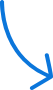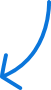days

hrs

mins

secs

See The Deals »

# What Is A PolyrhythmA polyrhythm is a combination of two or more rhythms played simultaneously while moving at the same linear tempo. So generally speaking, two rhythms are considered a polyrhythm if the number one is their only common divisor. In this free drum lesson, Dave Atkinson explains what’s a polyrhythm and shows you how a polyrhythm sounds on the drum set.

Each polyrhythm is named after a formula that lets you know exactly how the different rhythms relate to each other. The formula is the following: X-over-Y or X:Y. X is called the counter rhythm and Y is called the basic pulse. For helping you develop a good understanding of how this formula works, we’ll take a look at two practical examples and methodologies (A and B) for coming up with polyrhythms, by using a 3-over-4 polyrhythm as our test subject.

### Polyrhythms – Step 1: Setting A Number Of Counts/ Bars & The Basic Pulse

Example A: Use Y as the number of counts over which you’ll play the polyrhythm, before its various rhythms line up on the following count 1. Since on a 3-over-4 polyrhythm Y is 4, the polyrhythm will have to be played over four counts. For demonstration purposes, we notated the basic pulse on the bass drum line from the sheet music below.Example B: Alternatively, you can use Y as the number of bars over which you play the polyrhythm, before its various rhythms line up on a count 1. Since Y is 4, the polyrhythm has to be played over four bars before it loops. The methodology followed on example B is actually the approach we’ll be following during this whole series of free drum lessons on polyrhythms.### Polyrhythms – Step 2: Subdividing Each Count/ Bar With X

Example A: The X tells us exactly in how many notes we’ll have to subdivide each count to play the counter rhythm. Because X is 3 in a 3-over-4 polyrhythm, each count will be subdivided into three notes, that is, into 8th note triplets. The 8th note triplets are notated on the hi-hat line from the sheet music below.Example B: If you used Y to set the number of bars, X will define the number of counts per bar and the number of notes you’ll subdivide each count with, to play the counter rhythm. Since X is 3, each bar has three counts that are subdivided into 8th note triplets. This means we’ll have four bars of 3/4 odd-time.### Polyrhythms – Step 3: Setting The Counter Rhythm Over The Basic Pulse

Finally, for either example, repeat a rhythm each Yth note of the 8th note triplets. This is the counter rhythm to the basic pulse set on step 1. Taking a look at the sheet music below, you can see that we used a one-note counter rhythm for example A, which is played on each 4th note.The counter rhythm can have more than a single note per Y notes, as does the basic pulse. So you can actually play around with different ideas using the same polyrhythm – you’re not bound to a single polyrhythmic pattern. You can find an example of this by taking a look at the sheet music below, where we set the counter rhythm of example B as the first two 8th note triplets. As you can see, the rhythm repeats every set of 4 notes as well.Now that you understand how polyrhythms work, it’s easier to understand exactly why an X-over-Y polyrhythm is actually quite different from a Y-over-X polyrhythm. If you still don’t understand why they’re different, repeat the three-step process we just provided you with on a 4-over-3 polyrhythm instead.

Before you start learning how to practice and apply different polyrhythms to your drumming, we encourage you to check the free drum lesson “How To Read A Polyrhythm Chart” first. In that free drum lesson you’ll be instructed on how to read and write notation with the Time Unit Box System (TUBS). This is a useful notation system for showcasing and simplifying the relationships between complex rhythms, which would be harder to understand with traditional music notation otherwise.

•Yavar Askari says:

Your examples are very tight, nice drumming! However, to be fair, I’m pretty sure that polyrhythms involve different tuplet values played simultaneously – isn’t what you refer to as polyrhythms actually polymetric drumming? Correct me if I’m wrong!

•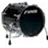Cadence says:

Yay Ive been looking for a site like this its awesome. Not to mention well organized, thoroughly informative, and quite helpful. :-).

•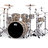tophei says:

very impressive…nice lesson..

•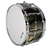eduardo sanpedro says:

I realy like it ,its tricki, fun and sounds good.

•Rafat says:

Yes.﻿ Use your wrists and frgiens as much as possible. I use my arms for fun because I move a lot when i get excited (live especially) but i can play just using my wrist with very little arm movement.

•Zuilma says:

Its impressing for me to see this videos because i already knew this without having a teacher so i guess i keep on watching this videos to learn how every drum lesson it’s called :p